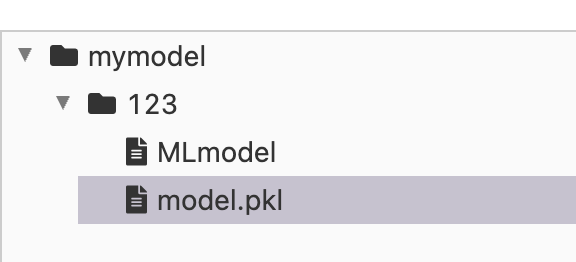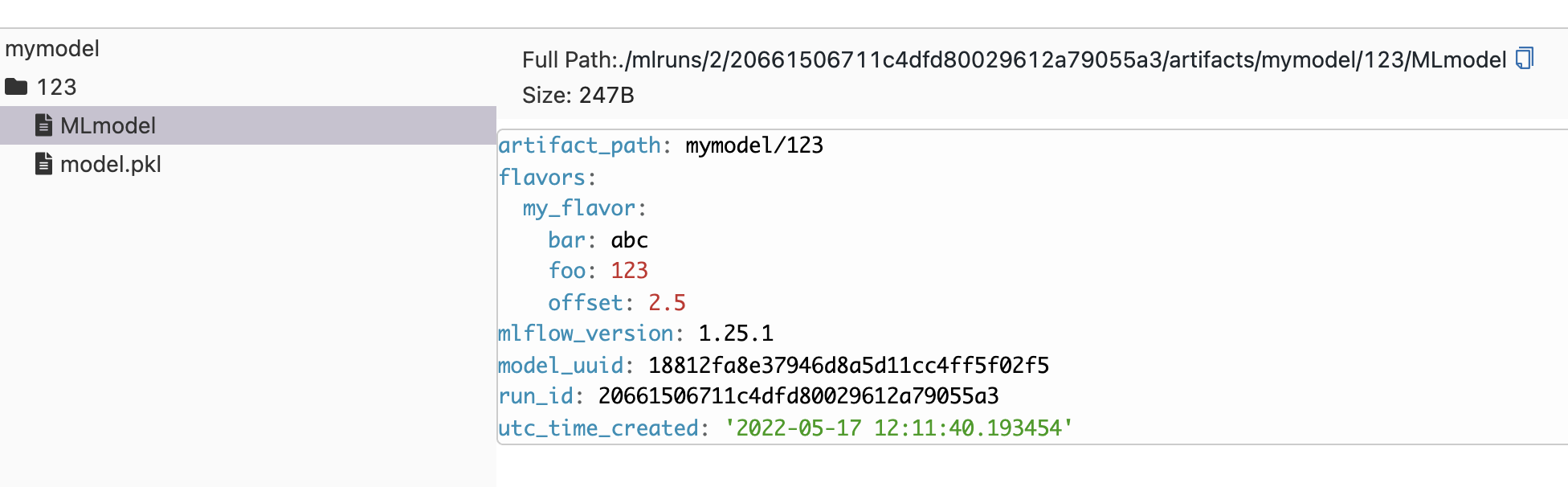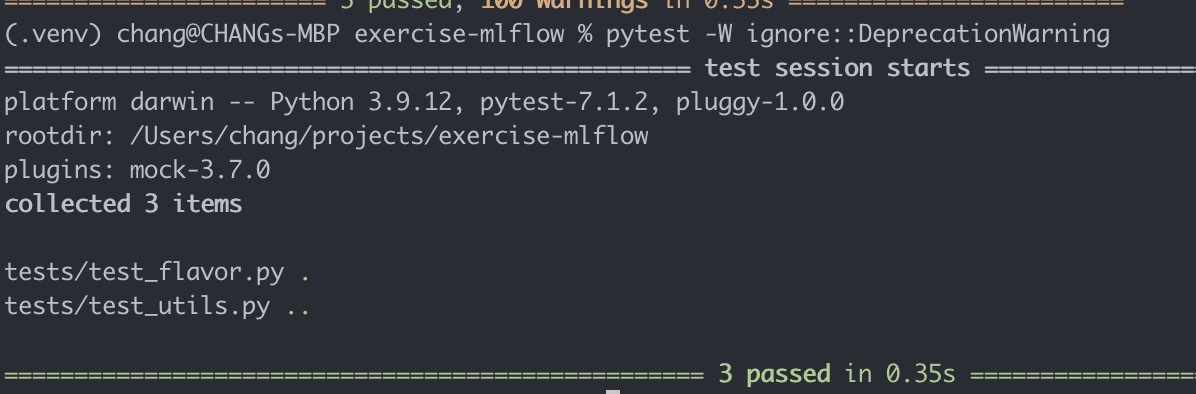# Create your own MLFlow custom flavors for model registry

A minimal tutorial on how to write your own MLFlow flavor code to save, log, load models with tests.

## MLFlow Model Registry

MLFlow is great for managing the machine learning model deployment lifecycle. But soon I ran into the question of “What if my model isn’t supported by MLFlow?” In particular, to register a model to the Model Registry, you must call `mlflow.<flavor>.log_model()` – but what if the flavor does not exist?

In this post, I will show you how to write a custom flavor code with tests that extends the list of MLFlow supported flavors so you can save ANY model to MLFlow.

## Example code

I put all my example code in this post in the repository:

• https://github.com/changhsinlee/mlflow-flavor-example

with the caveat that I did not set up the Model Registry backend in the repo.

## The code

There are three functions that you need to create in order to make MLFlow work:

• `save_model()` - Implementation of model specific saving methods
• `log_model()` - Registers model to Model Registry. Calls `save_model()` under the hood.
• `load_model()` - Loads model from Model Registry. This is mostly used at inference time.

Let’s go through each one of them.

When writing your custom flavor code, I recommend looking into how other flavors are implemented in the official repo. It will help you decide how much you need to implement for your own use case.

For example,

### What’s in the code

For demonstration purpose, instead of using a real ML library like `huggingface`, I’m using a fake object called `FakeModel()` here that has `save()`, `load()`, and `predict()` methods

``````import pickle
from pathlib import Path

class FakeModel:
def __init__(self, offset):
self.offset = offset

def __eq__(self, other):
return self.offset == other.offset

def predict(self, input_num: float):
return input_num + self.offset

def save(self, path):
pickle.dump(self, Path(path).open(mode="wb"))

@classmethod
``````

My goal is that, if I run

``````model = FakeModel(3)
# assuming this model is registered to Model Registry
log_model(model, artifact_path=artifact_directory)
``````

then I should be able to pass the following test

``````# assuming model_uri points to the model I saved above
assert 7 == loaded_model.predict(4)  # 3 + 4 = 7
``````

You can replace the `FakeModel` with a heavy hitter model but the general code pattern will be the same.

Let’s go into the three functions.

### Function 1. `save_model()`

This one is the longest.

You can view the source code for this part on GitHub for better formatting.

``````from mlflow.models import Model
from mlflow_flavor_example.utils import FakeModel
from mlflow.models.model import MLMODEL_FILE_NAME
from pathlib import Path

FLAVOR_NAME = "my_flavor"

def save_model(
fake_model: FakeModel,
path,
mlflow_model=None,
):

path = Path(path).resolve()
path.mkdir(parents=True, exist_ok=True)

mlflow_mlmodel_file_path = path / MLMODEL_FILE_NAME
model_subpath = path / "model.pkl"
if mlflow_model is None:
mlflow_model = Model()
mlflow_model.save(mlflow_mlmodel_file_path)
fake_model.save(model_subpath)
``````

In this example, `fake_model` is the only argument that points to a model object. But you can actually put as many objects here as you want. For NLP, you may need both the model and the tokenizer. Or you may want to save both the encoder and decoder for an autoencoder separately. The key here is that you need to decide how your model/tokenizer/encoder should be saved with their respective methods because it determines how they are loaded in `load_model()`.

``````model_subpath = path / "model.pkl"
# you will see this line's counterpart in load_model()
fake_model.save(model_subpath)
``````

The path `model_subpath` determines where the model weight is saved in the artifacts folder.We also need to save an artifact called `MLModel`:MLFlow uses this file to store flavor metadata related to the model and we can implement it by

``````from mlflow.models import Model
from mlflow.models.model import MLMODEL_FILE_NAME

if mlflow_model is None:
mlflow_model = Model()
mlflow_model.save(mlflow_mlmodel_file_path)
``````

The `foo`, `bar`, `offset` in the `add_flavor()` call here can be any metadata that you want to log or load at inference time.

### Function 2. `log_model()`

You can view the source code for this part on GitHub for better formatting.

``````from mlflow_flavor_example.utils import FakeModel
import mlflow_flavor_example

def log_model(
model: FakeModel,
artifact_path,
**kwargs,
):
return Model.log(
artifact_path=str(artifact_path),  # must be string, numbers etc
flavor=mlflow_flavor_example.flavor,  # points to this module itself
fake_model=model,
**kwargs,
)
``````

This function is a short wrapper around `save_model()`. The only part that was confusing to me was that I need to set

``````flavor=<name_of_the_module>.flavor
``````

despite the fact that I did not declare `flavor` anywhere in the module. Here, name_of_the_module is `mlflow_flavor_example` that I imported at the top. Because this is a MLFlow specific interface, I just follow the convention.

### Function 3. `load_model()`

You can view the source code for this part on GitHub for better formatting.

``````from mlflow.tracking.artifact_utils import _download_artifact_from_uri

artifact_uri=model_uri, output_path=dst_path
)
model_subpath = Path(local_model_path) / "model.pkl"
``````

This one is also short. It use the utility `_download_artifact_from_uri()` provided by MLFlow which downloads the artifact folder, whose structure we defined in `save_model()`, and load the model files based on the corresponding methods from how we saved the files in `save_model()`

Note that there are two things I didn’t explore here:

1. How do I pull metadata out of the flavor file `MLModel`? I think this is a good example from the spaCy flavor:

`````` from mlflow.utils.model_utils import _get_flavor_configuration

flavor_conf = _get_flavor_configuration(model_path=local_model_path, flavor_name=FLAVOR_NAME)
spacy_model_file_path = os.path.join(local_model_path, flavor_conf.get("data", "model.spacy"))
``````

Basically, I can use the `_get_flavor_configuration()` utility which loads `MLModel` as a dictionary into `flavor_conf`, then grab the values from said dictionary.

2. What if I have more than one model weights to load? If you save multiple things in `save_model()`, then it makes sense to load all of them here and return a `Tuple`. An example would be returning both `tokenizer` and `model` for an NLP model.

### Testing

Let’s write a test for them. I use `pytest`.

The single most useful test here, in my opinion, is an integration test that tests both `save_model()` and `load_model()`. You can see the example here:

• https://github.com/changhsinlee/mlflow-flavor-example/blob/main/tests/test_flavor.py

The question here becomes how do we set up the tests without calling a remote MLFlow server. Fortunately, the MLFlow team has it set up, so I copied the `conftest.py` from the MLFlow test suite and deleted parts that I don’t need:

• https://github.com/changhsinlee/mlflow-flavor-example/blob/main/tests/conftest.py

Under the hood, the fixtures here spin up an in-memory SQLite instance to act as temporary MLFlow backend. So when you run this integration test, it is as if you are calling a real MLFlow server. This is a better testing strategy than mocking so I am happy to keep it this way. To run the tests, I can use `pytest -W ignore::DeprecationWarning` to suppress `DeprecationWarnings`.You can add other tests by looking at other flavor’s source code.

## What else you can do

If you compare the code here with the MLFlow flavor code in the official repository, you can see that I skipped quite a lot of stuff:

• I didn’t touch `autolog()` at all since I don’t use this pattern at work or for my personal projects.
• I don’t like using `pyfunc()`.
• No `requirements.txt` or `conda` files: I believe this is better taken cared of at the project setup level. If the run environment comes with`git`, then MLFlow Experiment can track the git hash. So one should be able to reproduce the results if `requirements.txt` or other project dependency files like `pyproject.toml` is part of the repository anyway. A huge chunk of the official source code is about dealing with dependencies but I didn’t need this.
• No Signature or Examples: this depends on how you use MLFlow. I didn’t need this either.

and you can pick and choose what you want to do with your own use cases.

Written on May 18, 2022